# COSC 3340 Introduction to Theory of Computation University

• Slides: 23
Download presentation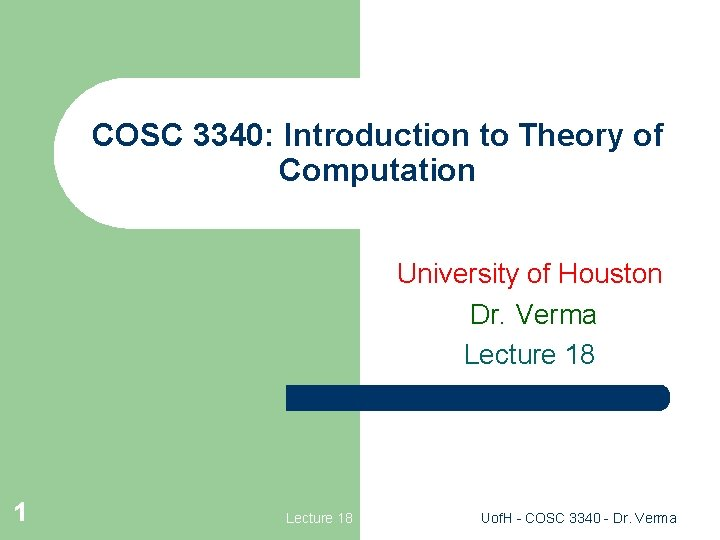COSC 3340: Introduction to Theory of Computation University of Houston Dr. Verma Lecture 18 1 Lecture 18 Uof. H - COSC 3340 - Dr. VermaCombining Turing Machines l l l A Turing Machine can be a component of another Turing Machine (“subroutine”) We will develop a graphical notation to build larger machines for more complex tasks easily. The scheme is hierarchical. Combination is possible since all TM’s are designed to be “nonhanging” – so the first machine can save something on the leftend of the tape. Reference: “Elements of the Theory of Computation’’ by H. R. Lewis and C. H. Papadimitriou. 2 Lecture 18 Uof. H - COSC 3340 - Dr. Verma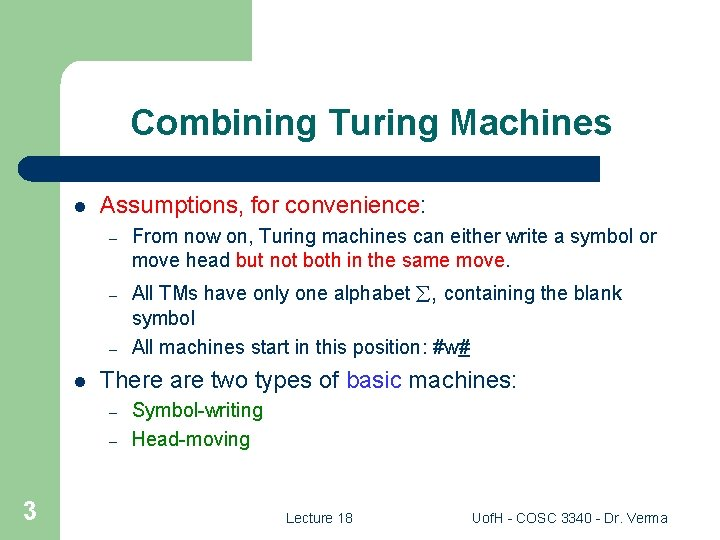Combining Turing Machines l Assumptions, for convenience: – From now on, Turing machines can either write a symbol or move head but not both in the same move. – All TMs have only one alphabet , containing the blank symbol All machines start in this position: #w# – l There are two types of basic machines: – – 3 Symbol-writing Head-moving Lecture 18 Uof. H - COSC 3340 - Dr. Verma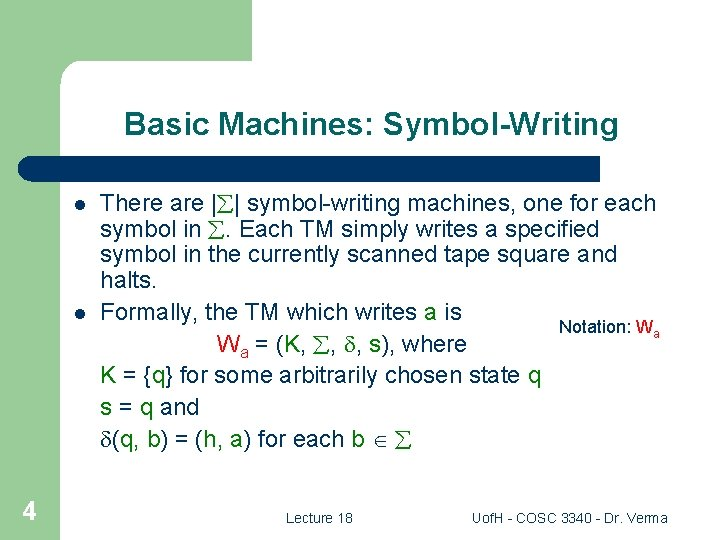Basic Machines: Symbol-Writing l l 4 There are | | symbol-writing machines, one for each symbol in . Each TM simply writes a specified symbol in the currently scanned tape square and halts. Formally, the TM which writes a is Notation: Wa Wa = (K, , , s), where K = {q} for some arbitrarily chosen state q s = q and (q, b) = (h, a) for each b Lecture 18 Uof. H - COSC 3340 - Dr. Verma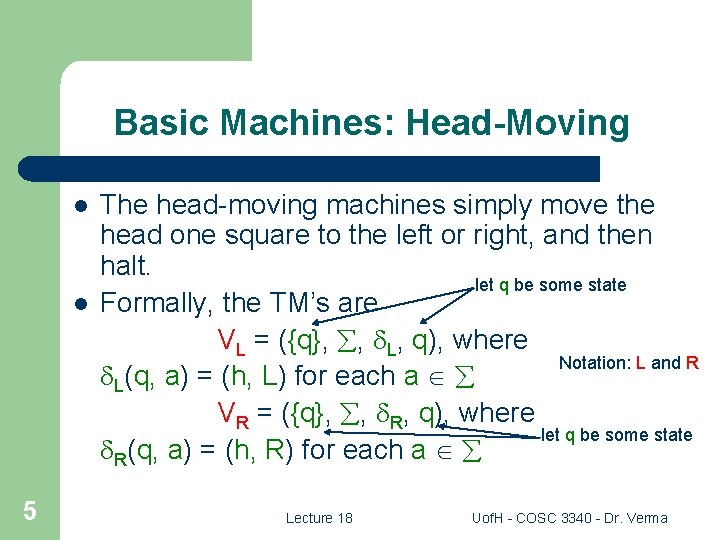Basic Machines: Head-Moving l l 5 The head-moving machines simply move the head one square to the left or right, and then halt. let q be some state Formally, the TM’s are VL = ({q}, , L, q), where Notation: L and R L(q, a) = (h, L) for each a VR = ({q}, , R, q), where let q be some state R(q, a) = (h, R) for each a Lecture 18 Uof. H - COSC 3340 - Dr. VermaRules for Combining Machines l l 6 Machines may be connected just like a Finite Automaton. If two machine are connected, then the first machine has to halt before the other machine starts. Lecture 18 Uof. H - COSC 3340 - Dr. Verma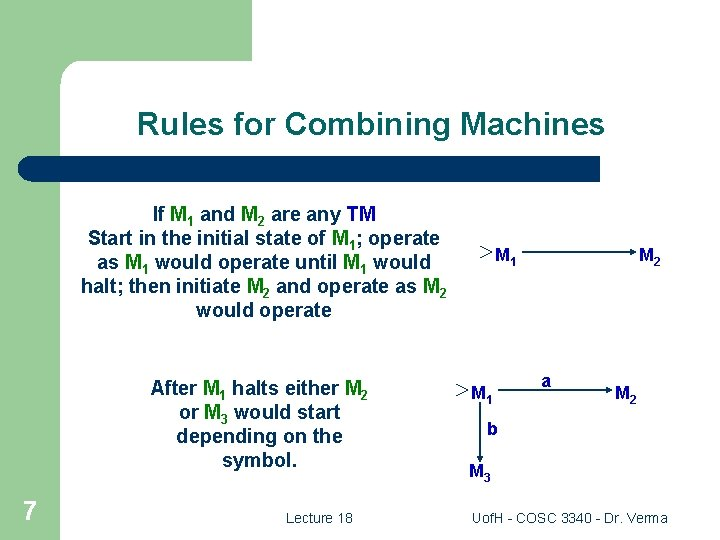Rules for Combining Machines If M 1 and M 2 are any TM Start in the initial state of M 1; operate as M 1 would operate until M 1 would halt; then initiate M 2 and operate as M 2 would operate After M 1 halts either M 2 or M 3 would start depending on the symbol. 7 Lecture 18 M 1 M 2 a M 2 b M 3 Uof. H - COSC 3340 - Dr. Verma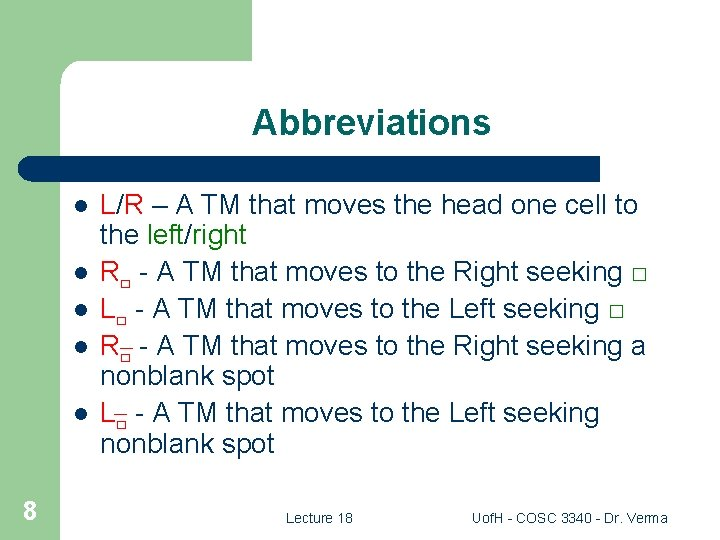Abbreviations l l l 8 L/R – A TM that moves the head one cell to the left/right R□ - A TM that moves to the Right seeking □ L□ - A TM that moves to the Left seeking □ R□ - A TM that moves to the Right seeking a nonblank spot L□ - A TM that moves to the Left seeking nonblank spot Lecture 18 Uof. H - COSC 3340 - Dr. VermaExample: R□ Alphabet ( ) = {a, b} M = ({q 0, q 1 }, {a, b, □}, , q 0, {}) a R□ R 9 b State Symbol Next state action q 0 □ (q 1, □, R) q 0 a (q 1, a, R) q 0 b (q 1, b, R) q 1 □ halt q 1 a (q 1, a, R) q 1 b (q 1, b, R) L□ is very similar to R□ Lecture 18 Uof. H - COSC 3340 - Dr. VermaJFLAP SIMULATION 10 Lecture 18 Uof. H - COSC 3340 - Dr. VermaJFLAP SIMULATION 11 Lecture 18 Uof. H - COSC 3340 - Dr. VermaJFLAP SIMULATION 12 Lecture 18 Uof. H - COSC 3340 - Dr. Verma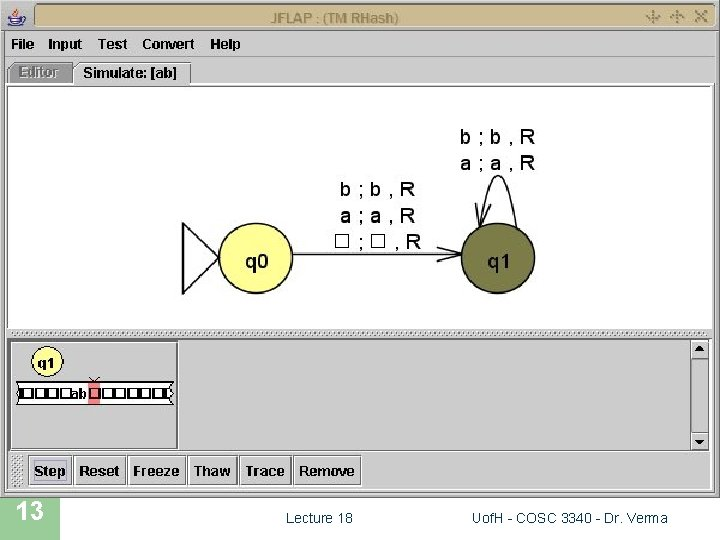JFLAP SIMULATION 13 Lecture 18 Uof. H - COSC 3340 - Dr. VermaJFLAP SIMULATION 14 Lecture 18 Uof. H - COSC 3340 - Dr. Verma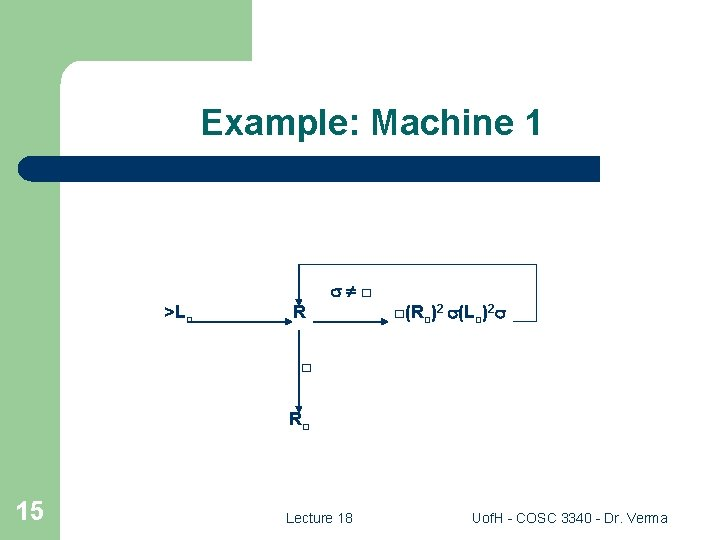Example: Machine 1 □ >L□ R □(R□)2 (L□)2 □ R□ 15 Lecture 18 Uof. H - COSC 3340 - Dr. Verma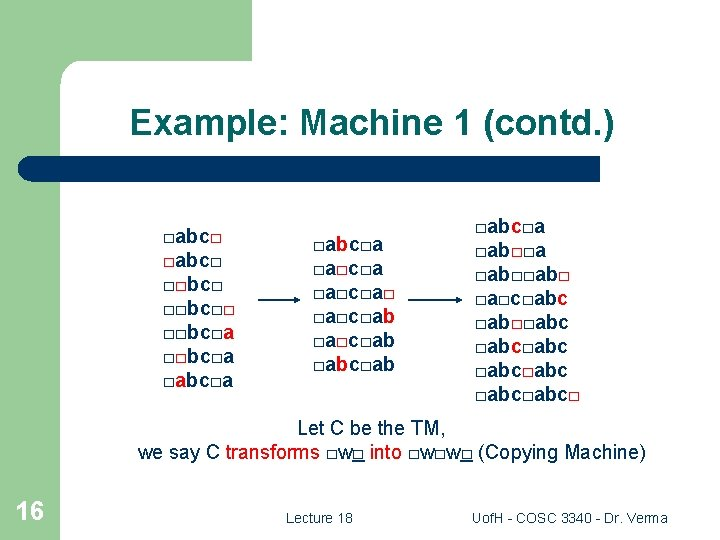Example: Machine 1 (contd. ) □abc□ □□bc□□ □□bc□a □abc□a □a□c□a□ □a□c□ab □abc□ab □abc□a □ab□□ab□ □a□c□abc □ab□□abc□abc□ Let C be the TM, we say C transforms □w□ into □w□w□ (Copying Machine) 16 Lecture 18 Uof. H - COSC 3340 - Dr. VermaExample: Machine 2 □ >L□ R L R □ L□ 17 Lecture 18 Uof. H - COSC 3340 - Dr. Verma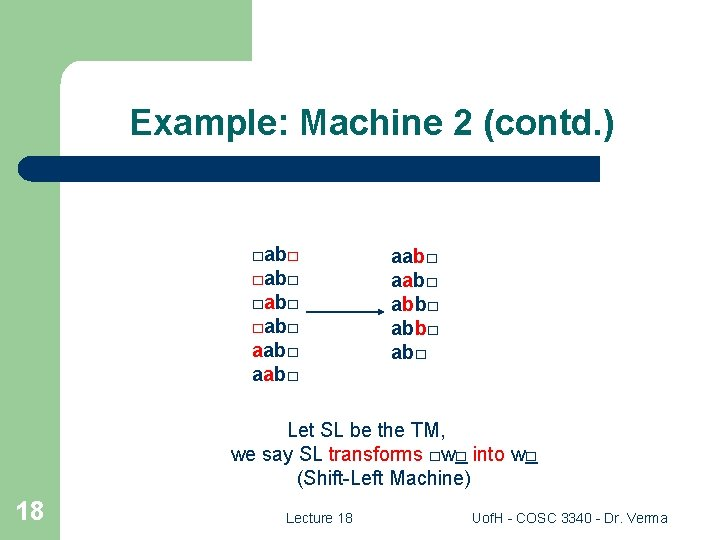Example: Machine 2 (contd. ) □ab□ aab□ abb□ ab□ Let SL be the TM, we say SL transforms □w□ into w□ (Shift-Left Machine) 18 Lecture 18 Uof. H - COSC 3340 - Dr. Verma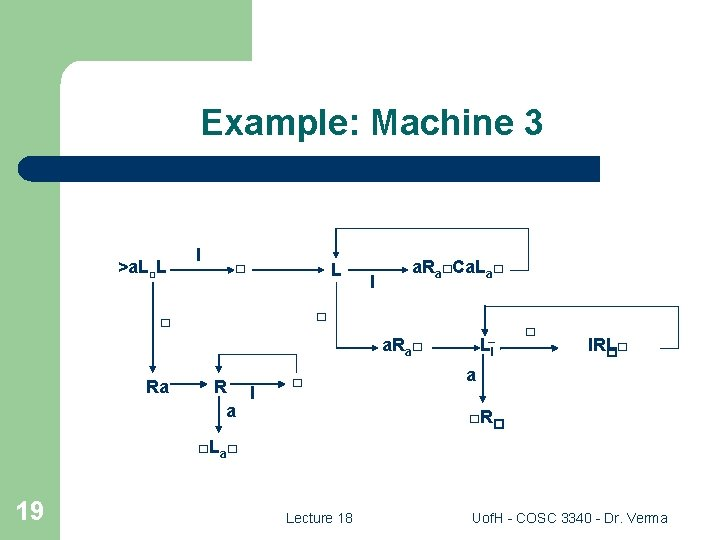Example: Machine 3 >a. L□L I □ L I a. Ra□Ca. La□ □ □ LI a. Ra□ Ra R I a □ □ IRL□ � a □R� □La□ 19 Lecture 18 Uof. H - COSC 3340 - Dr. VermaExample: Machine 3 (contd. ) □II□II□IIa □II□IIa □I□□IIa □a□□IIa □a□□II□II□ 20 □a□□II□IIa □□□□II□IIa a□□□II□IIa a□□□II□II□ a□□□IIIII□ a□□□IIII□□ a□□IIIII□□. . . □IIII□□□□□ f(2, 2) = ? f(n, m) = n m is computed by the TM (Multiplication Machine) Lecture 18 Uof. H - COSC 3340 - Dr. Verma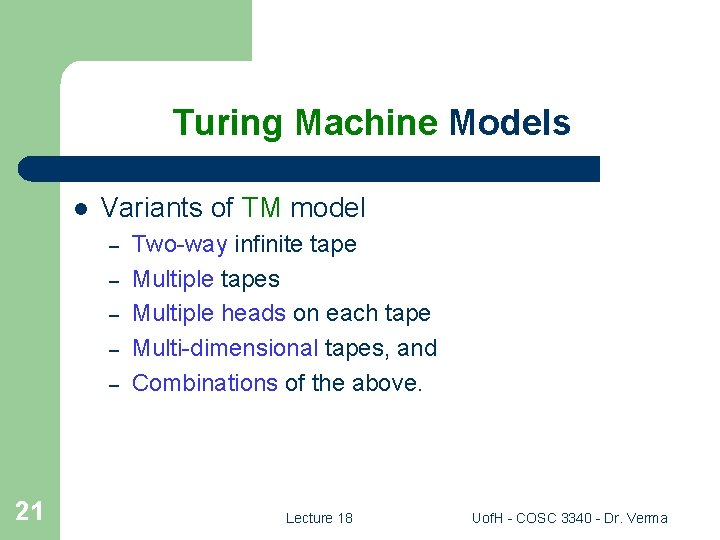Turing Machine Models l Variants of TM model – – – 21 Two-way infinite tape Multiple tapes Multiple heads on each tape Multi-dimensional tapes, and Combinations of the above. Lecture 18 Uof. H - COSC 3340 - Dr. Verma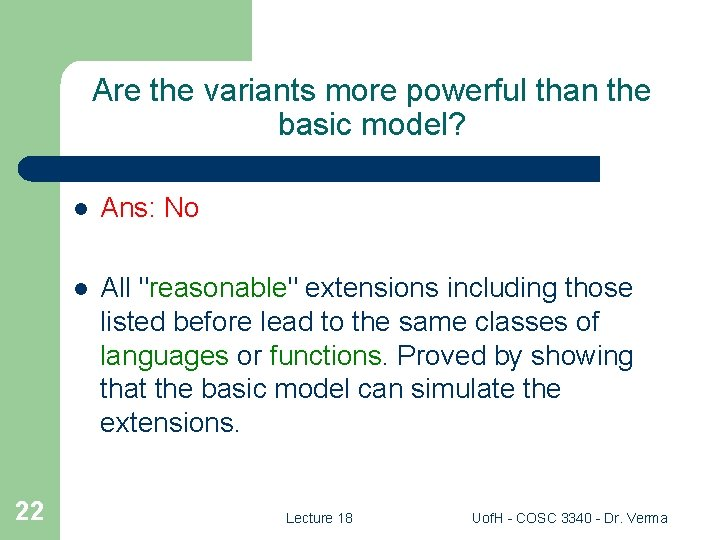Are the variants more powerful than the basic model? 22 l Ans: No l All "reasonable" extensions including those listed before lead to the same classes of languages or functions. Proved by showing that the basic model can simulate the extensions. Lecture 18 Uof. H - COSC 3340 - Dr. VermaTechniques for Simulations l The basic TM has – – expanded set of states expanded alphabet Note: one step in extended model is usually simulated by many steps in the basic model. 23 Lecture 18 Uof. H - COSC 3340 - Dr. Verma